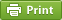Werner's Blog — Opinion, Analysis, Commentary
Two-sided market power in a vertical market

Vertical market structures come in all kinds of forms, but what many have in common is that there is market power involved. Consider a simple vertical market with an intermediate goods producer (I) and a final goods producer (F). The downstream producer (F) must buy intermediate goods from the upstream producer (I) according to a fixed input-output relationship. Think of it as an oil well and a refinery. The upstream producer cannot sell the crude oil to consumers without refining, and the downstream producer cannot sell gasoline to consumers without buying crude oil first. Both producers completely depend on each other. But who gets to keep the profits? The answer is quite different from the case of double marginalization where a retailer buys goods from a wholesaler, but it's the same good and ultimately the same market. With crude oil and gasoline, the relationship involves product transformation.

For the benefit of my students, below is a simplified model to illustrate how the profits can end up with either of the two producers depending on their bargaining power. The solution to this situation is called a Nash-in-Nash equilibrium because it involves two-sided bargaining between buyer and seller.

Consider a final-good market with negative exponential demand so that final good demand $$q$$ depends on the final-good price $$p$$ $q=a\cdot\exp(-p/b)$ where $$a$$ is zero-price demand and $$b$$ is the unit-elasticity price. Negative exponential demand is algebraically a little more elegant than linear demand, but this choice does not matter much here.

The final-good producer makes profits $\pi_F=(p-c_F)q$ while the intermediate-good producer makes profits $\pi_I=(r-c_I)s$ where final good $$q$$ and intermediate good $$s$$ are linked through the input-output relationship $q=\theta\cdot s$ This means that for every unit of the intermediate good we can produce $$\theta$$ units of the final product. The crucial element that links upstream and downstream producer is the intermediate-good price $$r$$. How is it determined?

First consider the case of vertical integration. In this case there is only a single decision maker. The internal transfer price between the upstream and downstream units is $$r=0$$, and thus the optimal solution is given by maximizing the joint profit function $\pi=(p-c_F-c_I/\theta)q$ The first-order condition for the profit maximum yields the solution for the equilibrium market price $p^\ast = b+c_F+c_i/\theta$ Thus, profits are $$\pi=bq^\ast$$. It turns out that regardless of the allocation of market power, the upstream and downstream producer will replicate the profit-maximizing market outcome. It is just the allocation of profits that will change.

Assume that the final-good producer has all the market power. In that case all profits accrue to that producer, and zero profits to the intermediate goods producer. The downstream firm is thus forced to sell the intermediate goods at cost so that $$r=c_I$$. Now switch around the market power and assume that the intermediate goods producer has all the power, whereas the final goods producer has none. It is easy to see that in this case the intermediate goods producer charges a price $$r=(p-c_F)\theta$$ to extract all the profits. At that intermediate-good price, the final-good producer breaks even but makes no profits.

Now we introduce market power into the bargaining process. Assume that the bargaining power is captured by a parameter $$\phi\in[0,1]$$ that characterizes the strength of the final-good producer, so that $$1-\phi$$ is the bargaining power of the intermediate-good producer. The Nash-in-Nash equilibrium, following Horn and Wolinksy (1988) is the solution to the maximization problem $\max_r\left\{ \pi_F(r)^\theta\cdot \pi_I(r)^{1-\theta} \right\}$ This is a simplified version of the equilibrium that assumes that bargaining failure leads to the outcomes without bargaining power. The first-order condition of this maximization problem yields the optimal intermediate-good price: $r^\ast = \phi c_I+(1-\phi)\theta(p-c_F)$ In other words, the intermediate-good price is a mixture of the two extreme outcomes that were shown earlier. It follows immediately that the profits are split so that $$\pi_F= \phi b q^\ast$$ and $$\pi_I = (1-\phi) b q^\ast$$. The bargaining power strength $$\phi$$ determines the allocation of profits between the upstream and downstream firm. This is a very intuitive result.

The above solution leaves unresolved what determines the bargaining power $$\phi$$ between the upstream and downstream firm. In the introductory example of an oil well and refinery, the two parties depend on each other, so there is no particular reason why one party has the upper hand. Often bargaining power is resolved in a dynamic setting, while the above solution is static. The two firms might as well vertically integrate as the market outcome will not change. If there is a significant cost to the bargaining process, integration is indeed economically sensible. Vertical integration of this monopsony-monopoly structure has no adverse effect on consumers. Of course, if markets are oligopolistic and there are more complex vertical structures, the picture gets much more complicated. The point of the above exercise was to show that two vertically connected markets are quite different from double marginalization in a product market.

Posted on Monday, February 7, 2022 at 09:00 — #EconomicsRecent Blog Entries

Topics

Months

Subscribe to RSS feed© 2023  Prof. Werner Antweiler, University of British Columbia.